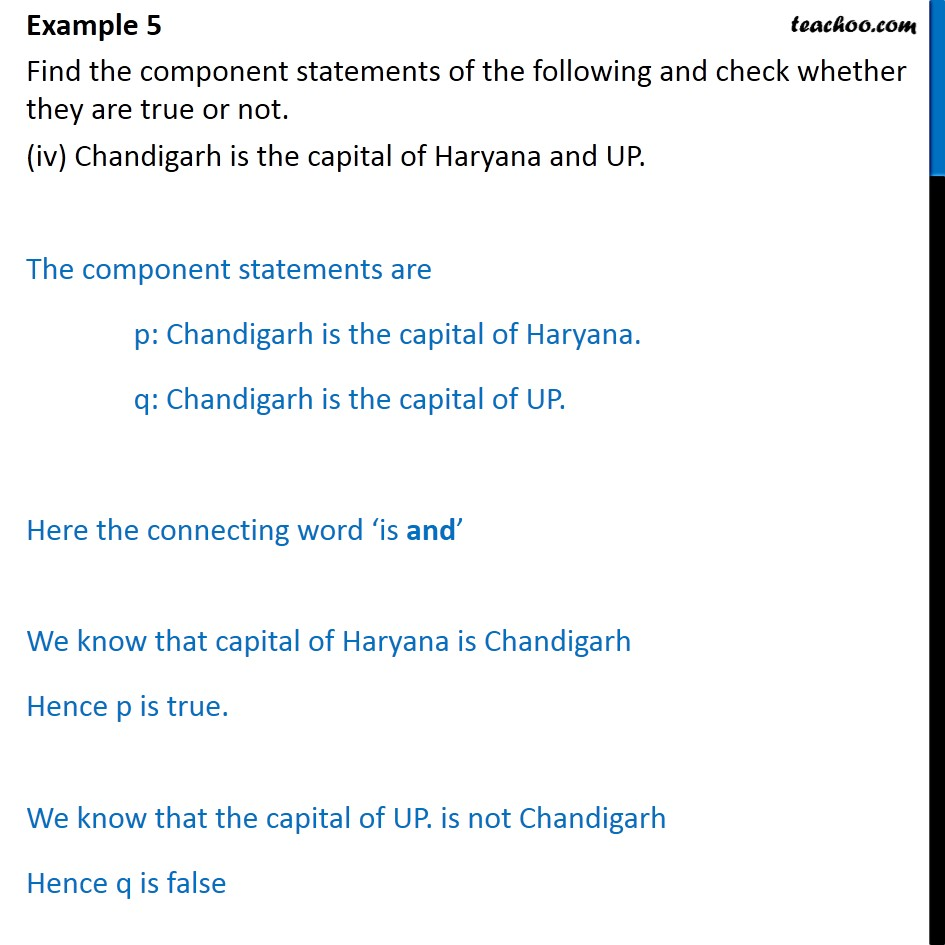1. Chapter 14 Class 11 Mathematical Reasoning (Deleted)
2. Serial order wise
3. Examples

Transcript

Example 5 Find the component statements of the following and check whether they are true or not. (iv) Chandigarh is the capital of Haryana and UP. The component statements are p: Chandigarh is the capital of Haryana. q: Chandigarh is the capital of UP. Here the connecting word is and We know that capital of Haryana is Chandigarh Hence p is true. We know that the capital of UP. is not Chandigarh Hence q is false

Examples

Chapter 14 Class 11 Mathematical Reasoning (Deleted)
Serial order wise